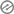# Financial Management MCQ on Cost of Capital

## MCQ on Cost of Capital1. The inter sources of finance in the form of retained earning of an organization involves

(a) Explicit cost

(b) Implicit cost

(c) Both of the above

(d) None of the above

2. The cost of capital encompasses

(a) Explicit cost

(b) Implicit cost

(c) Both of the above

(d) None of the above

3. The minimum rate of return that suppliers of capital expect as the compensation for their contribution of fund is known as

(a) Cost of capital

(b) Payoffs

(c) Both of the above(d) None of the above

4. Capital assets pricing model was introduced by

(a) William Shape(b) John Cina

(c) Jan Mossin

(d) Jan Morison

5. The analysis that estimate the beta on the basis of company growth, size, earnings, variability, leverage and industry affiliation is known as

(a) Cross sectional regression

(b) Accounting earing approach

(c) Fundamental information approach

(d) None of the above

`Answer (c) Fundamental information approach `

6. If there is rise in beta , then the expected return

(a) Decrease

(b) Increase

(c) Constant(d) None of the above

7. The external source of finance in the form of equity and debt involves

(a) Explicit cost

(b) Implicit cost

(c) Both of the above

(d) None of the above

8. The annual rate of return that an investor expects to earn from the investment in shares of a company is known as

(a) Cost of equity

(b) Cost of debt

(c) Cost of retaining earning

(d) None of the above

9. The important assumption of capital assets pricing model are

(a) All investor are price taker

(b) All investor are rational and risk averse

(c) All investor aim to maximize their economic utilities

(d) All of the above

10. The cost of capital is the cost from……………… Perspective?

(a) Fund raiser

(b) Fund provider

(c) Both of the above

(d) None of the above

11. The equality of expected rate of return of a security and market indicates that the betas is equal to

(a) 2

(b) 3

(c) 1

(d) 4